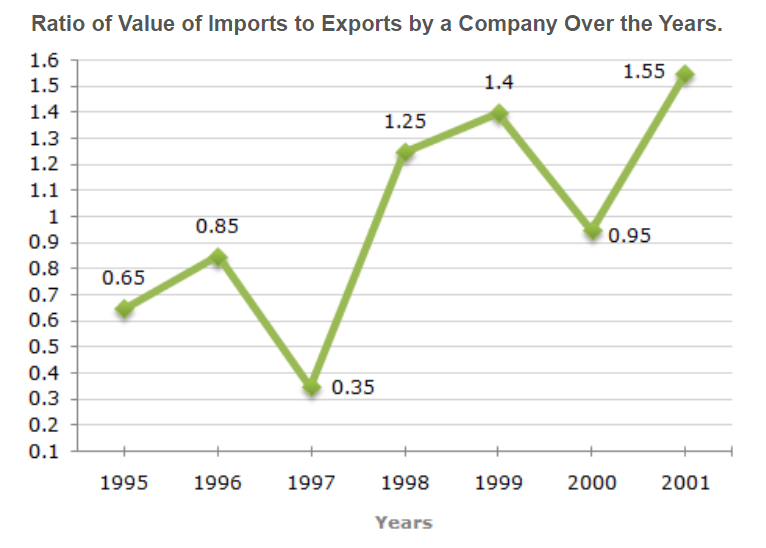# E-PolyLearning

 6. The following line graph gives the ratio of the amounts of imports by a company to the amount of exports from that company over the period from 1995 to 2001. If the imports in 1998 was Rs. 250 crores and the total exports in the years 1998 and 1999 together was Rs. 500 crores, then the imports in 1999 was ?a. Rs. 250 crores b. Rs. 300 crores c. Rs. 357 crores d. Rs. 420 crores

 7. The following line graph gives the ratio of the amounts of imports by a company to the amount of exports from that company over the period from 1995 to 2001. The imports were minimum proportionate to the exports of the company in the year ?a. 1995 b. 1996 c. 1997 d. 2000
 8. The following line graph gives the ratio of the amounts of imports by a company to the amount of exports from that company over the period from 1995 to 2001. What was the percentage increase in imports from 1997 to 1998 ?a. 72 b. 56 c. 28 d. Data inadequate
 9. The following line graph gives the ratio of the amounts of imports by a company to the amount of exports from that company over the period from 1995 to 2001. If the imports of the company in 1996 was Rs. 272 crores, the exports from the company in 1996 was ?a. Rs. 370 crores b. Rs. 320 crores c. Rs. 280 crores d. Rs. 275 crores
 10. The following line graph gives the ratio of the amounts of imports by a company to the amount of exports from that company over the period from 1995 to 2001. In how many of the given years were the exports more than the imports ?a. 1 b. 2 c. 3 d. 4